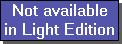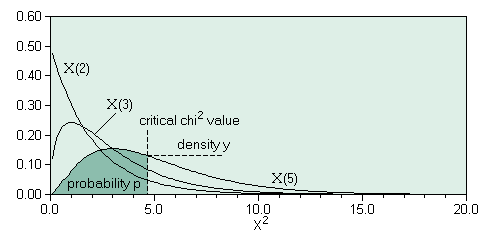The SDL Component Suite is an industry leading collection of components supporting scientific and engineering computing. Please visit the SDL Web site for more information....## Chi2DistriQuantileUnit: SDL_statis Class: none Declaration: function Chi2DistriQuantile (p: double; df: integer): double;

The function Chi2DistriQuantile returns the quantile value for the chi-square distribution for a given probability p and a given number of degrees of freedom df. The parameter p may take any value in the open interval (0,1). If Chi2DistriQuantile is called using an invalid value of p a value of 0.0 is returned.Hint 1: The calculated value is exact to about 8 significant figures.

 Hint 2: The function Chi2DistriQuantile calculates the quantile by using the integral from 0.0 to the c2 value. This differs from the convention of how the integral of the c2 distribution is used in many statistical packages, which tabulate the integral between +infinite and the c2 value. Thus you have to use 1-p to get comparable results. For example, in order to calculate the function FCHIINV(p,df) of Microsofts Excel® you have to use Chi2DistriQuantile(1-p,df).

Last Update: 2020-Aug-04